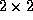Next: Regularizing effect in one-space Up: Approximate Solutions of Nonlinear Previous: References

# Kinetic Formulations and Regularity

### Abstract:

We discuss the kinetic formulation of nonlinear conservation laws and related equations, a kinetic formulation which describes both the equation and the entropy criterion. This formulation is a kinetic one, involving an additional variable called velocity by analogy. We apply this formulation to derive, based upon the velocity averaging lemmas, new compactness and regularity results. In particular, we highlight the regularizing effect of nonlinear entropy solution operators, and we quantify the gained regularity in terms of the nonlinearity. Finally, we show that this kinetic formulation is in fact valid and meaningful for more general classes of equations, including equations involving nonlinear second-order terms, and thehyperbolic system of isentropic gas dynamics, in both Eulerian or Lagrangian variables ( - the so called 'p-system').